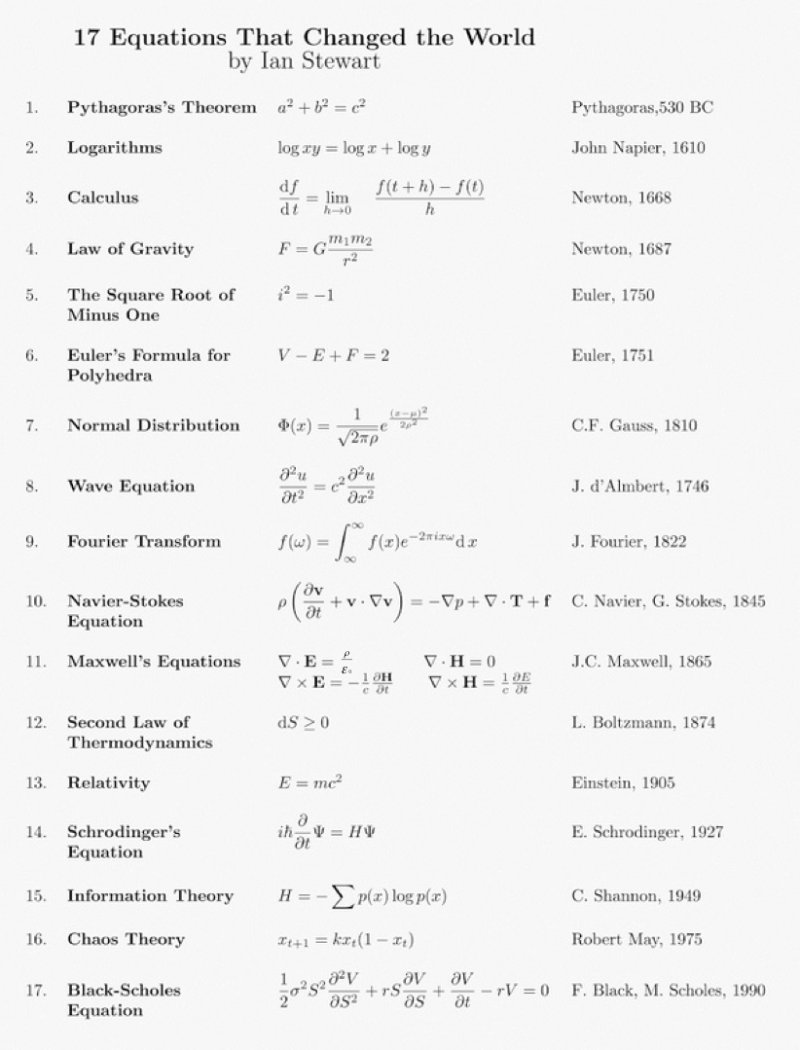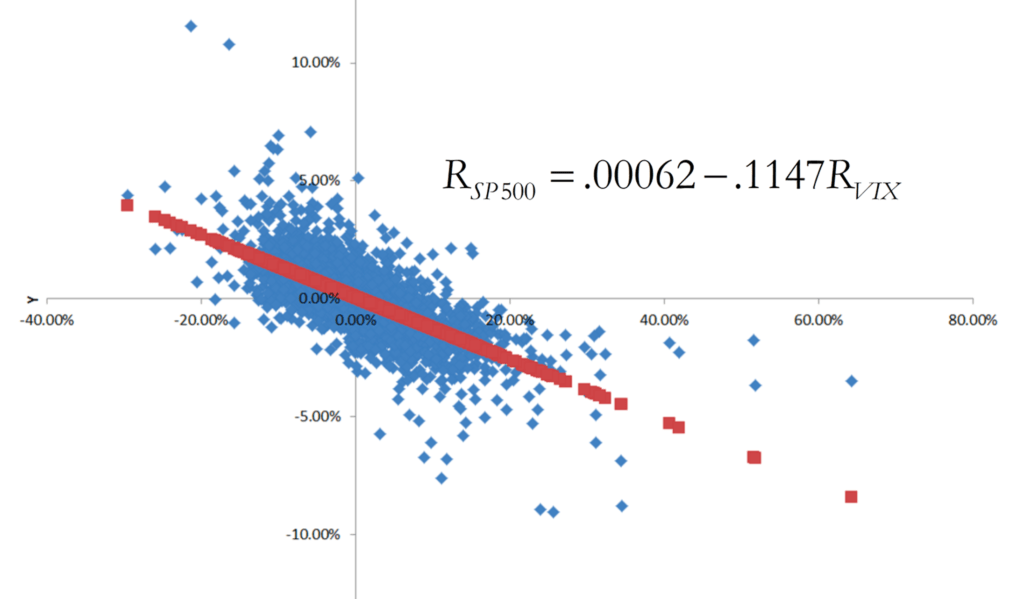## The 17 equations that changed the course of history (spoiler alert: we use 4 of these equations in Finance 4366!)

I especially like the fact that Ian Stewart includes the famous Black-Scholes equation (equation #17) on his list of the 17 equations that changed the course of history; Equations (2), (3), (7), and (17) play particularly important roles in Finance 4366!From Ian Stewart’s book, these 17 math equations changed the course of human history.

## Calculus, Probability and Statistics, and a preview of future topics in Finance 4366

Calculus (yesterday’s Finance 4366 class topic) and probability and statistics (next week’s Finance 4366 class topics) are foundational for the theory of pricing and managing risk with financial derivatives.

On Tuesday, January 31, we will introduce and describe the nature of financial derivatives, and motivate their study with examples of forwards, futures, and options. Derivatives are so named because they derive their values from one or more underlying assets. Underlying assets typically involve traded financial assets such as stocks, bonds, currencies, or other derivatives, but derivatives can derive value from pretty much anything. For example, the Chicago Mercantile Exchange (CME) offers exchange-traded weather futures and options contracts (see “Market Futures: Introduction To Weather Derivatives“). There are also so-called “prediction” markets in which derivatives based upon the outcome of political events are actively traded (see “Prediction Market“).

Besides introducing financial derivatives and discussing various institutional aspects of markets in which they are traded, we’ll consider various properties of forward and option contracts, since virtually all financial derivatives feature payoffs that are isomorphic to either or both schemes. For example, a futures contract is simply an exchange-traded version of a forward contract. Similarly, since swaps involve exchanges between counter-parties of payment streams over time, these instruments essentially represent a series of forward contracts. In the option space, besides traded stock options, many corporate securities feature “embedded” options; e.g., a convertible bond represents a combination of a non-convertible bond plus a call option on company stock. Similarly, when a company makes an investment, so-called “real” options to expand or abandon the investment at some future is often present.

Perhaps the most important (pre-Midterm 1) idea that we’ll introduce is the concept of a so-called “arbitrage-free” price for a financial derivative. While details will follow, the basic idea is that one can replicate the payoffs on a forward or option by forming a portfolio comprising the underlying asset and a riskless bond. This portfolio is called the “replicating” portfolio, since, by design, it replicates the payoffs on the forward or option. Since the forward or option and its replicating portfolio produce the same payoffs, then they must also have the same value. However, suppose the replicating portfolio (forward or option) is more expensive than the forward or option (replicating portfolio). If this occurs, then one can earn a riskless arbitrage profit by simply selling the replicating portfolio (forward or option) and buying the forward or option (replicating portfolio). However, competition will ensure that opportunities for riskless arbitrage profits vanish quickly. Thus the forward or option will be priced such that one cannot earn arbitrage profit from playing this game.

## On the relationship between the S&P 500 and the CBOE Volatility Index (VIX)

Besides going over the course syllabus during the first day of class on Tuesday, January 17, we will also discuss a particularly important “real world” example of financial risk. Specifically, we will study the relationship between realized daily stock market returns (as measured by daily percentage changes in the SP500 stock market index) and changes in forward-looking investor expectations of stock market volatility (as indicated by daily percentage changes in the CBOE Volatility Index (VIX)):As indicated by this graph (which also appears in the lecture note for the first day of class), daily percentage changes on closing prices for the SP500 (the y-axis variable) and for the VIX (the x-axis variable) are strongly negatively correlated with each other. The blue dots are based on 8,315 contemporaneous observations of daily returns for both variables, spanning the 33-year period of time starting on January 2, 1990 and ending on December 30, 2022. When we fit a regression line through this scatter diagram, we obtain the following equation:${R_{SP500}} = .00062 - .1147{R_{VIX}}$,

where${R_{SP500}}$ corresponds to the daily return on the SP500 index and${R_{VIX}}$ corresponds to the daily return on the VIX index. The slope of this line (-0.1147) indicates that on average, daily closing SP500 returns are inversely related to daily closing VIX returns.  Furthermore, nearly half of the variation in the stock market return during this time period (specifically, 48.87%) can be statistically “explained” by changes in volatility, and the correlation between${R_{SP500}}$ and${R_{VIX}}$ came out to -0.70. While a correlation of -0.70 does not imply that daily closing values for${R_{SP500}}$ and${R_{VIX}}$ always move in opposite directions, it does suggest that this will be the case more often than not. Indeed, closing daily values recorded for${R_{SP500}}$ and${R_{VIX}}$ during this period moved inversely 78.59% of the time.